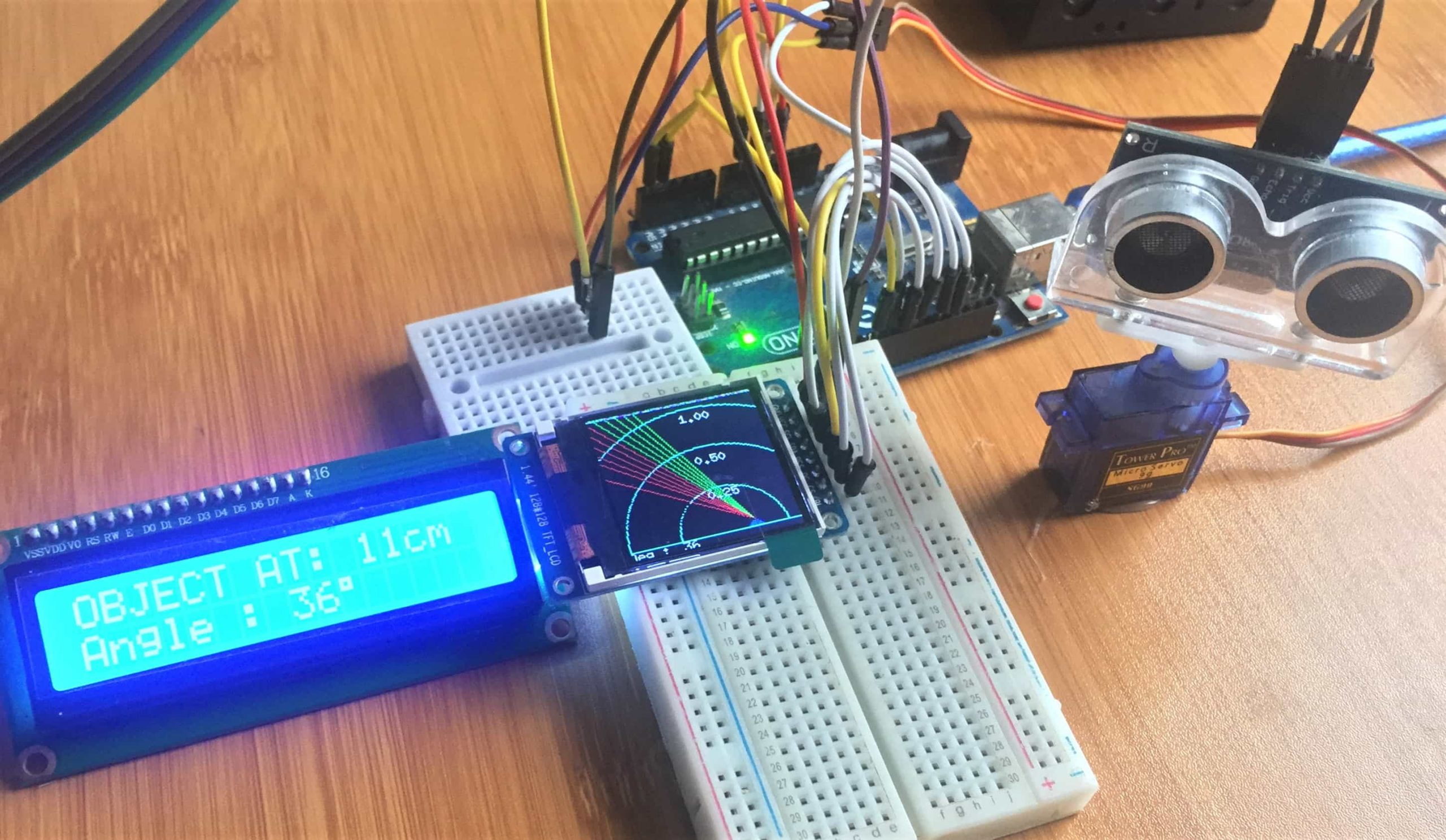# Simple Ultrasonic Radar System using Arduino.Ultrasonic radar systems have a number of applications in object detection especially in water navigation. Though this is called a radar but actually it’s a sonar system due to the mode of operation which involves ultrasonic transmitters sending a sound wave generated from a piezo electric transducer, to the media being measured. The device measures the length of time it takes for the reflected sound wave to return to the transducer.

In another of my post i described in detail the working of the HC-SR04 ultrasonic sensor which is the major component in this setup. So before you proceed you can first check out the working of this sensor from the link :

• Measuring distance using HC-SR04 Ultrasonic sensor and Arduino.
• We also include a stepper motor for rotating the sensor, an I2C lcd display and a ST3755 TFT Color display. You can also review the interfacing of these devices with Arduino by visiting these posts:

• How to connect 16×2 I2C lcd display with Arduino.
• How to control a servo motor with Arduino.
• Using the 1.44″ TFT ST7735 Color display with Arduino.
• ## Schematic for the Ultrasonic radar using Arduino and HC-SRO4 sensor.

For the simple Ultrasonic radar we are making,t he hc-sr04 ultrasonic sensor, the servo motor, 16×2 LCD display and ST7735 TFT Color display are connected to the Arduino board as shown above. The pin connections for the ultrasonic sensor and the TFT display are done as illustrated in the table below.

For the servo motor, the signal line is connected to Arduino pin 3. The SDA and SCL lines of the I2C LCD are connected to Arduino pin A4 and A5 respectively.

## Code for the Ultrasonic Radar using Arduino.

``````#include <SPI.h>
#include <Wire.h>
#include <LiquidCrystal_I2C.h>
#include <Servo.h>.
#include "Ucglib.h"

const int trigPin = 6;
const int echoPin = 5;
int Ymax = 128;
int Xmax = 128;
int base = 8;
int pos = base+6;
int deg=0;
int x;
int val =200;
int j = 2;
Servo myServo;
LiquidCrystal_I2C lcd(0x27, 2, 1, 0, 4, 5, 6, 7, 3, POSITIVE);

long duration;
int distance;
int k;

Ucglib_ST7735_18x128x160_SWSPI ucg(/*sclk=*/ 13,
/*data=*/ 11, /*cd=*/ 9, /*cs=*/ 10, /*reset=*/ 8);

void setup(void)
{
delay(1000);
myServo.write(80);
lcd.begin(16,2); // Initialiser l'interface de Lcd avec leurs Dimensions
lcd.backlight();

ucg.begin(UCG_FONT_MODE_SOLID);
ucg.setFont(ucg_font_6x10_tr);
ucg.clearScreen();
ucg.setRotate270();

pinMode(trigPin, OUTPUT); // Sets the trigPin as an Output
pinMode(echoPin, INPUT); // Sets the echoPin as an Input
Serial.begin(9600);
myServo.attach(3); // Defines on which pin is the servo motor attached

}

void loop(void)
{

fix();

for ( x=80; x >= 10; x--){
distance = calculateDistance();
Serial.println(distance);
k = map(x, 80, 10, 15,165);
myServo.write(k);

if (distance < 20){
int f = x+6;
ucg.setColor(0, 0, 255); //color when object is detected
lcd.setCursor(0,0);
lcd.print("OBJECT AT: ");
lcd.print(distance);
lcd.print("cm  ");
delay(10);
lcd.setCursor(0,1);
lcd.print("Angle : ");
lcd.print(deg);
lcd.print((char)223);
lcd.print("  ");
delay(2000);
}
else{
lcd.setCursor(0,0);
lcd.print("NOTHING IN RANGE");
delay(10);
lcd.setCursor(0,1);
lcd.print("Angle : ");
lcd.print(deg);
lcd.print((char)223);
lcd.print("  ");
delay(80);
}
ucg.setColor(0, 207, 0);

int d = x+1;
ucg.setColor(0, 207, 0);

int c = x+2;
ucg.setColor(0, 207, 0);

int b = x+3;
ucg.setColor(0, 102, 0);

int a = x+4;
ucg.setColor(0, 102, 0);

int e = x+5;
ucg.setColor(0, 0, 0);
//background color
ucg.setColor(220, 220, 220); //color of horizontal labels
ucg.setPrintPos(160,0);
ucg.setPrintDir(2);
ucg.print("Deg :");
deg = map (x, 80, 10 , 0, 180);
ucg.setPrintPos(120,0);
ucg.setPrintDir(2);
ucg.print(deg);
ucg.setPrintPos(10,0);
ucg.print(distance);

ucg.setColor(220, 220, 220); //colors of vertical axis (RED)
ucg.setPrintPos(90,38);
ucg.setPrintDir(2);
ucg.print("0.25");
ucg.setPrintPos(90,70);
ucg.print("0.50");
ucg.setPrintPos(90,110);
ucg.print("1.00");
}

fix();

for ( x=10; x <= 80; x++){
distance =
calculateDistance();
Serial.println(distance);
k = map(x, 10, 80, 165,15);
myServo.write(k);
if (distance < 20){
int e = x-5;
ucg.setColor(0, 0, 255);//color when object is detected
lcd.setCursor(0,0);
lcd.print("OBJECT AT: ");
lcd.print(distance);
lcd.print("cm  ");
delay(10);
lcd.setCursor(0,1);
lcd.print("Angle : ");
lcd.print(deg);
lcd.print((char)223);
lcd.print("  ");
delay(80);
}
else{
lcd.setCursor(0,0);
lcd.print("NOTHING IN RANGE");
delay(10);
lcd.setCursor(0,1);
lcd.print("Angle: ");
lcd.print(deg);
lcd.print((char)223);
lcd.print("  ");
delay(80);
}

ucg.setColor(0, 207, 0);

int a = x-1;

int b = x-2;
ucg.setColor(0, 102, 0);

int c = x-3;
ucg.setColor(0, 102, 0);

int d = x-4;
ucg.setColor(0, 0, 0); //background color
ucg.setColor(220, 220, 220);// color or horizontal labels
ucg.setPrintPos(160,0);
ucg.setPrintDir(2);
ucg.print("Deg :");
deg = map (x, 10, 80 , 0, 180);
ucg.setPrintPos(120,0);
ucg.setPrintDir(2);
ucg.print(deg);
ucg.setPrintPos(10,0);
ucg.print(distance);

ucg.setColor(220, 220, 220); //color of vertical axis labels
ucg.setPrintPos(90,38);
ucg.setPrintDir(2);
ucg.print("0.25");
ucg.setPrintPos(90,70);
ucg.print("0.50");
ucg.setPrintPos(90,110);
ucg.print("1.00");

}

}

void fix(){
ucg.setColor(255, 0, 0);
ucg.drawDisc(Xmax/2, base, 5, UCG_DRAW_LOWER_RIGHT);
ucg.drawDisc(Xmax/2, base, 5, UCG_DRAW_LOWER_LEFT);

ucg.setColor(225, 255, 50);// (YELLOW)
ucg.drawCircle(80, base, 115,
UCG_DRAW_LOWER_RIGHT);
ucg.drawCircle(80, base, 115, UCG_DRAW_LOWER_LEFT);

ucg.drawCircle(80, base, 78, UCG_DRAW_LOWER_RIGHT);
ucg.drawCircle(80, base, 78, UCG_DRAW_LOWER_LEFT);

ucg.drawCircle(80, base, 40, UCG_DRAW_LOWER_RIGHT);
ucg.drawCircle(80, base, 40, UCG_DRAW_LOWER_LEFT);

ucg.drawLine(0, base, 160,base);

ucg.setColor(0, 0, 0);
ucg.drawBox(100, 0, 30, 8);
}

int calculateDistance(){
digitalWrite(trigPin, LOW);
delayMicroseconds(2);
// Sets the trigPin on HIGH state for 10 micro seconds
digitalWrite(trigPin, HIGH);
delayMicroseconds(10);
digitalWrite(trigPin, LOW);
duration = pulseIn(echoPin, HIGH); // Reads the echoPin, returns the sound wave travel time in microseconds
distance= duration*0.034/2;
return distance;
}

``````

When this code is uploaded to the Arduino the servo motor will begin rotating clockwise and anticlockwise for 180 degrees in both directions. The TFT display shows the movement of the sensor using green lines and detection of an object using red lines.

The distance and angle of location of the object is displayed on the I2C LCD.
This system can be improved by adding an alarm and LEDs to give physical signals when an object is detected.